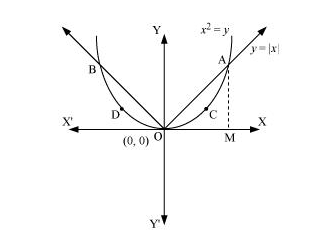# Find the area of the region bounded by the parabola

Question:

Find the area of the region bounded by the parabola $y=x^{2}$ and $y=|x|$

Solution:

The area bounded by the parabola, $x^{2}=y$, and the line, $y=|x|$, can be represented asThe given area is symmetrical about y-axis.

∴ Area OACO = Area ODBO

The point of intersection of parabola, $x^{2}=y$, and line, $y=x$, is $\mathrm{A}(1,1)$.

Area of $\mathrm{OACO}=$ Area $\triangle \mathrm{OAM}-$ Area OMACO

Area of $\Delta \mathrm{OAM}=\frac{1}{2} \times \mathrm{OM} \times \mathrm{AM}=\frac{1}{2} \times 1 \times 1=\frac{1}{2}$

Area of OMACO $=\int_{0}^{1} y d x=\int_{0}^{1} x^{2} d x=\left[\frac{x^{3}}{3}\right]_{0}^{1}=\frac{1}{3}$

$\Rightarrow$ Area of $\mathrm{OACO}=$ Area of $\triangle \mathrm{OAM}-$ Area of $\mathrm{OMACO}$

$=\frac{1}{2}-\frac{1}{3}$

$=\frac{1}{6}$

Therefore, required area $=2\left[\frac{1}{6}\right]=\frac{1}{3}$ units